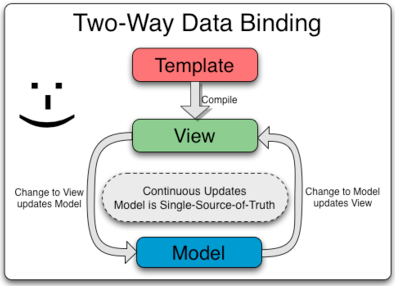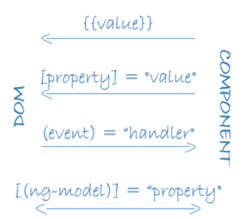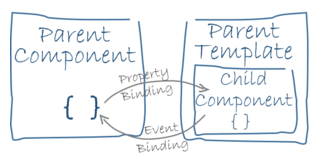# Data Binding to Accommodate Component Relationships

### Angular 1 bragged about two-way data binding. However, Angular 2 decided to promote one-way data binding (like ReactJS), and two-way data binding is considered only as a special case. Read on for more information.

· Web Dev Zone · Analysis
Save
2.32K Views

this article represents concepts and related code samples in relation with data binding in angular 2 vis-a-vis component relationships. those with prior experience in reactjs (which propagates one-way binding) should find it easy to understand. angular 1 bragged about two-way data binding; however, angular 2 decided to promote one-way data binding (like reactjs), and two-way data binding is considered only as a special case.

## two-way data binding in angularjs 1

let's look at how data binding looks in angular 1. it was termed as two-way data binding with two-way signifying following:

• any change in the model object would get updated on view.
• any change in the view would update the model object which would then update the view.

the diagram depicts two-way data binding in angular 1.for example, in the sample code below, there is an input element with ng-model assigned to "name". and, then, the "name" is accessed/displayed elsewhere in the view using the syntax such as {{name}}. see the code such as <h1>hello, {{name}}</h1>. with two-way data binding, any change in the input field will update the view with changed value.

``````<body ng-app="helloapp" ng-controller="helloctrl">
<h1>hello, {{name}}</h1>
<hr/>
<form>
<div>
<input type="text" ng-model="name">
</div>
</form>
<script type="text/javascript">
angular.module('helloapp', [])
.controller('helloctrl', ['\$scope', function(\$scope){
\$scope.name = "calvin";
}])
</script>
</body>``````

## data binding in angular 2

the diagram depicts the two-way data binding in angular 2.take a look at the following code:

``````@component({
selector: 'hello'
directives: [usercomponent]
})
@view({
template = `
<h1>hello, {{name}}</h1>
<form>
<div>
</div>
</form>
`
})
export class hellocomponent {
name = 'calvin hobbes';
count = 1;

updatecount = function() {
this.count++;
};

}``````

and now, here is what the code for "usercomponent" would look like. note the usage of "inputs: [‘username’]" for making the username as input property.

``````@component({
selector: 'user',
})
@view({
template: `
`
})
export class usercomponent {
}``````

in the diagram and code shown above, note the following:

• property binding : this is one-way data binding. the component property name is bound to the target element property. thus, the value from the component property flows to the target element. this is achieved using syntax such as:
• {{expression}}
• [target]=”expression”
• bind-target=”expression”
the above expressions are used to update the data from the component (data source) to the html view. with interpolation, the property is put into the template with double curly braces such as {{name}} in the code shown below.
• event binding : this is one-way data binding. the event binding (change) calls the updatecount method when the user writes something in the input field. the event binding is represented using the expression: (event) = "statement" or on-event="statement". this expression means that if the user input leads to an event, the application state is updated based on "statement" which could be a method/api of the component. in simple words, with the above expression the application state is updated based on the user input. the event occurring with the target element is used to update the component state.
• property & event binding (two-way data binding) : the syntax  [(ng-model)]="name" combines property and event binding in a single notation using the ngmodel directive. generally speaking, two-way data binding is represented using expressions such as [(target)] = "expression" or bindon-target="expression". the change made to the input field flows to the component thereby resetting the value as in event binding, and the change to name in component flows to the input field as in property binding. the diagram depicts this.in the same way, with parent-child relationships, the changes made in child component’s template flows to parent component as in event binding, and changes made in property of parent component flows to the child component as in property binding.• property binding for parent-child relationship : syntax such as [username]= "name" is used to bind the username property with parent’s component property where name should be an object. in this case, the username is required to be bound to interpolation string, the syntax would look like following: username = {{name}}.
Topics:
angular 2, data binding

Published at DZone with permission of Ajitesh Kumar, DZone MVB.

Opinions expressed by DZone contributors are their own.